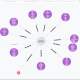## Graph Database – project Tycho with RNeo4j

Before I continue with R, I discovered another issue. When I started to process the data sets I obtained the Lat, Lon for each of the cities. I have used the spatial component of Neo4j before in a Java application. I was disappointed to discover that py2Neo doesn’t support this yet. The Neo4j Python REST Client does have support. Since spatial indexes can be added to existing data its not a big deal.

Having read Nicole White’s writings on Neo4j(http://nicolewhite.github.io/) and R I was excited to see how R works with Neo4j.

R and Neo4j
Having used R for spatial analysis I was curious to see what could be done with R and the Tycho data. I have yet to add spatial indexes but there is still a lot of other operations that don’t need spatial.

I am using R-Studio. RNeo4j can be found at http://nicolewhite.github.io/RNeo4j/. The instructions for installation  work well.  Following the examples given with the install I was able to run a simple query and obtain a data frame.

library(RNeo4j)
create a connection
`graph = startGraph("http://localhost:7474/db/data)"`
This just tells me that the connection is good.
graph\$version

Define a query. Here I am using one that just returns the list of states.
The first time I tried to run the query just as it was in Python.
`query = "MATCH (n:`State`) RETURN n"`

Error in as.data.frame.list(value, row.names = rlabs) :
supplied 54 row names for 1 rows

Nicole was quick to point out that to use Cypher to return nodes you should use getNodes(). For relations use getRels().
The new query: `"MATCH (n:State) RETURN n.description"`

Now running the query there is no error and I get a list of states.
`df = cypher(graph, query)`
Display the results

n.description
1 CA
2 GA
3 CT
4 AK
5 AL
6 CO
7 DC
and so on

The query `"MATCH (n:Case) RETURN n.year" `returns too much data to print out. Instead I filtered the data to a date range,n.year > 1900 and n.year 1900 and n.year < 1950 RETURN n.year,count(*) as count”
Looking at rows of data is okay but what I really wanted was something visual.
Again, Nicole’s site has examples of plotting. I started with plot().
This took a bit of experimenting to get it right.

`plot(df,xlab="year",ylab="count",main="CASES counts, all states, between 1900 and 1950" ) `This is more like it. What else is there?
ggplot() ?

The command below took a while to get correct. The aes() function wasn’t clear, but an explanation from here(http://docs.ggplot2.org/0.9.3/aes.html) helped. There are enough examples already that explain ggplot that I am not going into details.

```ggplot(df, aes(x = n.year, y =count))+geom_bar(stat = "identity",fill = "darkblue") + coord_flip() +labs(x = "Year", y = "Count", title = "Count of MEASLES over the entire range") +theme(axis.text = element_text(size = 12, color = "black"), axis.title = element_text(size = 14, color = "black"), plot.title = element_text(size = 16, color = "black")) ```With my confidence up, time to try something more interesting, more R like.
The query below returns all cases of the disease Measles. I want to try and fit a linear model to the results.

query = `"match (d:Disease)<-[:CASE_OF]-(dt:Case)--(ct:City)(st:State) where d.description ="MEASLES" return dt.year,count(*) as count" `Fitting a linear model to the data.
`model = lm(formula=count ~ dt.year, data=df) `
And then draw the “Line of Best Fit”
`abline(model) `It is interesting to see the differences between the states. Most of the queries showed a peak around the 1920’s and then a decline. I have found some information that indicates a peak or elevated number of cases reported in the 1920’s. The data would match that. What seems to be up for discussion is why. I am not an epidemiologist so I won’t go any further.